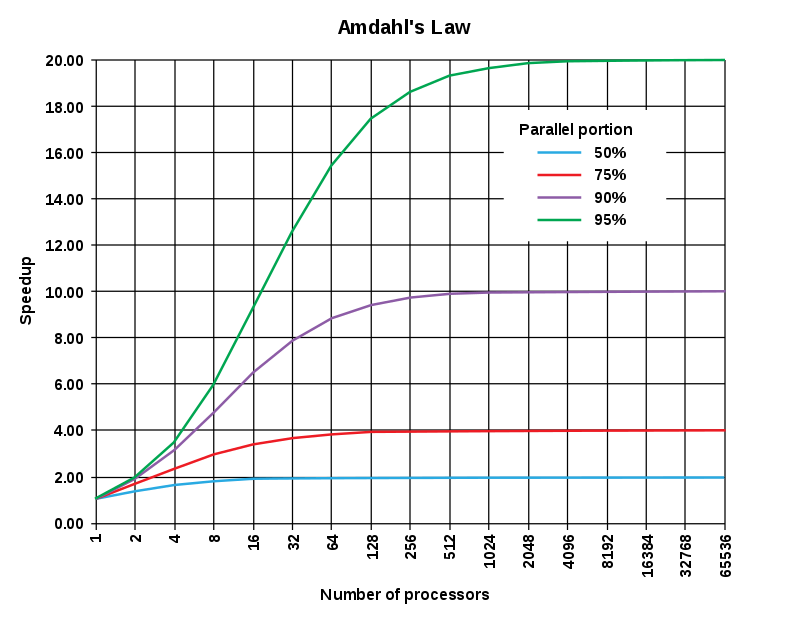# Amdahl’s law

Amdahl’s law，又叫做阿姆达尔定律，其意义在于表示了计算机并行计算处理任务的加速的极限。

## 简略证明

\$\$
T = (1-p)T + pT
\$\$

\$\$
T’ = (1-p)T + frac p s T
\$\$

\$\$
S_{text{latency}}(s) = frac {T} {T’} = frac{1}{1 – p + frac p s}
\$\$

## 意义

Amdahl’s law揭示了不管如何的进行并行计算，只要任务包含无法被并行加速的部分，那么加速比是有上限的，因为

\$\$
lim_{s rightarrow infty} S_{text{latency}}(s) = frac {T} {T’} = frac{1}{1 – p}
\$\$